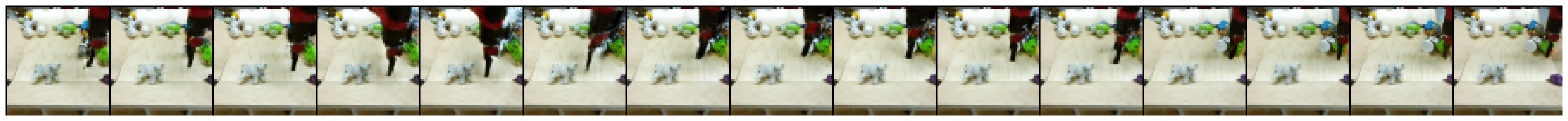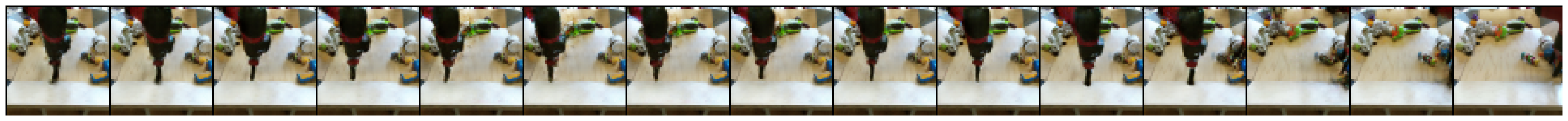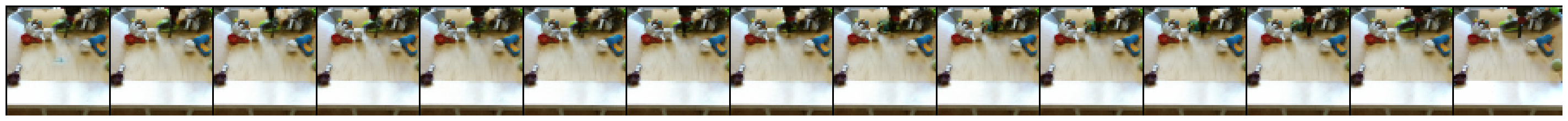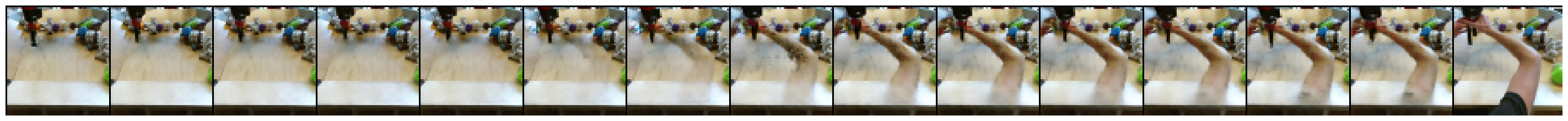# Video Inbetweening using 3D Convolutions

Yunpeng Li, Dominik Roblek, and Marco Tagliasacchi. From Here to There: Video Inbetweening Using Direct 3D Convolutions, 2019.

https://arxiv.org/abs/1905.10240

Current Hub characteristics:

• has models for BAIR Robot pushing videos and KTH action video dataset (though this colab uses only BAIR)
• BAIR dataset already available in Hub. However, KTH videos need to be supplied by the users themselves.
• only evaluation (video generation) for now
• batch size and frame size are hard-coded

## Setup

Since tfds.load('bair_robot_pushing_small', split='test') would download a 30GB archive that also contains the training data, we download a separated archive that only contains the 190MB test data. The used dataset has been published by this paper and is licensed as Creative Commons BY 4.0.

import tensorflow as tf

import matplotlib.pyplot as plt
import numpy as np
import seaborn as sns
import tensorflow_hub as hub
import tensorflow_datasets as tfds

from tensorflow_datasets.core import SplitGenerator
from tensorflow_datasets.video.bair_robot_pushing import BairRobotPushingSmall

import tempfile
import pathlib

TEST_DIR = pathlib.Path(tempfile.mkdtemp()) / "bair_robot_pushing_small/softmotion30_44k/test/"

# Download the test split to $TEST_DIR mkdir -p$TEST_DIR
wget -nv https://storage.googleapis.com/download.tensorflow.org/data/bair_test_traj_0_to_255.tfrecords -O \$TEST_DIR/traj_0_to_255.tfrecords

2020-10-02 12:40:49 URL:https://storage.googleapis.com/download.tensorflow.org/data/bair_test_traj_0_to_255.tfrecords [189852160/189852160] -> "/tmp/tmpd30jtu05/bair_robot_pushing_small/softmotion30_44k/test/traj_0_to_255.tfrecords" 


# Since the dataset builder expects the train and test split to be downloaded,
# patch it so it only expects the test data to be available
builder = BairRobotPushingSmall()
test_generator = SplitGenerator(name='test', gen_kwargs={"filedir": str(TEST_DIR)})
builder._split_generators = lambda _: [test_generator]

Downloading and preparing dataset bair_robot_pushing_small/2.0.0 (download: 30.06 GiB, generated: Unknown size, total: 30.06 GiB) to /home/kbuilder/tensorflow_datasets/bair_robot_pushing_small/2.0.0...
WARNING:tensorflow:From /home/kbuilder/.local/lib/python3.6/site-packages/tensorflow_datasets/video/bair_robot_pushing.py:106: tf_record_iterator (from tensorflow.python.lib.io.tf_record) is deprecated and will be removed in a future version.
Instructions for updating:
Use eager execution and:
tf.data.TFRecordDataset(path)

Warning:tensorflow:From /home/kbuilder/.local/lib/python3.6/site-packages/tensorflow_datasets/video/bair_robot_pushing.py:106: tf_record_iterator (from tensorflow.python.lib.io.tf_record) is deprecated and will be removed in a future version.
Instructions for updating:
Use eager execution and:
tf.data.TFRecordDataset(path)

Shuffling and writing examples to /home/kbuilder/tensorflow_datasets/bair_robot_pushing_small/2.0.0.incomplete346H0N/bair_robot_pushing_small-test.tfrecord
Dataset bair_robot_pushing_small downloaded and prepared to /home/kbuilder/tensorflow_datasets/bair_robot_pushing_small/2.0.0. Subsequent calls will reuse this data.



## BAIR: Demo based on numpy array inputs

# @title Load some example data (BAIR).
batch_size = 16

ds = builder.as_dataset(split="test")
test_videos = ds.batch(batch_size)
first_batch = next(iter(test_videos))
input_frames = first_batch['image_aux1'][:, ::15]
input_frames = tf.cast(input_frames, tf.float32)

WARNING:tensorflow:From /home/kbuilder/.local/lib/python3.6/site-packages/tensorflow_datasets/core/features/feature.py:334: calling map_fn_v2 (from tensorflow.python.ops.map_fn) with back_prop=False is deprecated and will be removed in a future version.
Instructions for updating:
results = tf.map_fn(fn, elems, back_prop=False)
Use:

Warning:tensorflow:From /home/kbuilder/.local/lib/python3.6/site-packages/tensorflow_datasets/core/features/feature.py:334: calling map_fn_v2 (from tensorflow.python.ops.map_fn) with back_prop=False is deprecated and will be removed in a future version.
Instructions for updating:
results = tf.map_fn(fn, elems, back_prop=False)
Use:

Warning:tensorflow:From /tmpfs/src/tf_docs_env/lib/python3.6/site-packages/tensorflow/python/util/deprecation.py:574: calling map_fn_v2 (from tensorflow.python.ops.map_fn) with dtype is deprecated and will be removed in a future version.
Instructions for updating:

Warning:tensorflow:From /tmpfs/src/tf_docs_env/lib/python3.6/site-packages/tensorflow/python/util/deprecation.py:574: calling map_fn_v2 (from tensorflow.python.ops.map_fn) with dtype is deprecated and will be removed in a future version.
Instructions for updating:


# @title Visualize loaded videos start and end frames.

print('Test videos shape [batch_size, start/end frame, height, width, num_channels]: ', input_frames.shape)
sns.set_style('white')
plt.figure(figsize=(4, 2*batch_size))

for i in range(batch_size)[:4]:
plt.subplot(batch_size, 2, 1 + 2*i)
plt.imshow(input_frames[i, 0] / 255.0)
plt.title('Video {}: First frame'.format(i))
plt.axis('off')
plt.subplot(batch_size, 2, 2 + 2*i)
plt.imshow(input_frames[i, 1] / 255.0)
plt.title('Video {}: Last frame'.format(i))
plt.axis('off')

Test videos shape [batch_size, start/end frame, height, width, num_channels]:  (16, 2, 64, 64, 3)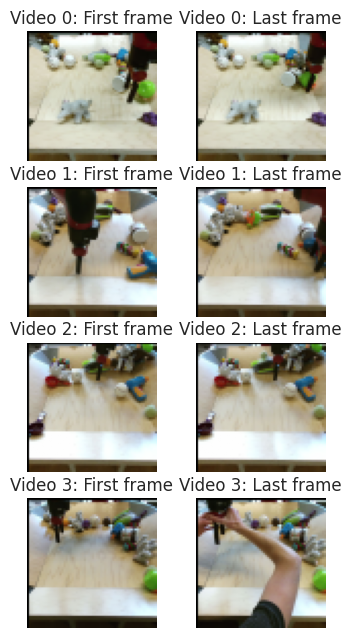hub_handle = 'https://tfhub.dev/google/tweening_conv3d_bair/1'

WARNING:tensorflow:Unable to create a python object for variable <tf.Variable 'video_discriminator/conv_0/weights:0' shape=(4, 4, 4, 3, 64) dtype=float32_ref> because it is a reference variable. It may not be visible to training APIs. If this is a problem, consider rebuilding the SavedModel after running tf.compat.v1.enable_resource_variables().

Warning:tensorflow:Unable to create a python object for variable <tf.Variable 'video_discriminator/conv_0/weights:0' shape=(4, 4, 4, 3, 64) dtype=float32_ref> because it is a reference variable. It may not be visible to training APIs. If this is a problem, consider rebuilding the SavedModel after running tf.compat.v1.enable_resource_variables().

Warning:tensorflow:Unable to create a python object for variable <tf.Variable 'video_discriminator/conv_0/LayerNorm/beta:0' shape=(64,) dtype=float32_ref> because it is a reference variable. It may not be visible to training APIs. If this is a problem, consider rebuilding the SavedModel after running tf.compat.v1.enable_resource_variables().

Warning:tensorflow:Unable to create a python object for variable <tf.Variable 'video_discriminator/conv_0/LayerNorm/beta:0' shape=(64,) dtype=float32_ref> because it is a reference variable. It may not be visible to training APIs. If this is a problem, consider rebuilding the SavedModel after running tf.compat.v1.enable_resource_variables().

Warning:tensorflow:Unable to create a python object for variable <tf.Variable 'video_discriminator/conv_0/LayerNorm/gamma:0' shape=(64,) dtype=float32_ref> because it is a reference variable. It may not be visible to training APIs. If this is a problem, consider rebuilding the SavedModel after running tf.compat.v1.enable_resource_variables().

Warning:tensorflow:Unable to create a python object for variable <tf.Variable 'video_discriminator/conv_0/LayerNorm/gamma:0' shape=(64,) dtype=float32_ref> because it is a reference variable. It may not be visible to training APIs. If this is a problem, consider rebuilding the SavedModel after running tf.compat.v1.enable_resource_variables().

Warning:tensorflow:Unable to create a python object for variable <tf.Variable 'video_discriminator/conv_1/weights:0' shape=(4, 4, 4, 64, 128) dtype=float32_ref> because it is a reference variable. It may not be visible to training APIs. If this is a problem, consider rebuilding the SavedModel after running tf.compat.v1.enable_resource_variables().

Warning:tensorflow:Unable to create a python object for variable <tf.Variable 'video_discriminator/conv_1/weights:0' shape=(4, 4, 4, 64, 128) dtype=float32_ref> because it is a reference variable. It may not be visible to training APIs. If this is a problem, consider rebuilding the SavedModel after running tf.compat.v1.enable_resource_variables().

Warning:tensorflow:Unable to create a python object for variable <tf.Variable 'video_discriminator/conv_1/LayerNorm/beta:0' shape=(128,) dtype=float32_ref> because it is a reference variable. It may not be visible to training APIs. If this is a problem, consider rebuilding the SavedModel after running tf.compat.v1.enable_resource_variables().

Warning:tensorflow:Unable to create a python object for variable <tf.Variable 'video_discriminator/conv_1/LayerNorm/beta:0' shape=(128,) dtype=float32_ref> because it is a reference variable. It may not be visible to training APIs. If this is a problem, consider rebuilding the SavedModel after running tf.compat.v1.enable_resource_variables().

Warning:tensorflow:Unable to create a python object for variable <tf.Variable 'video_discriminator/conv_0/weights:0' shape=(4, 4, 4, 3, 64) dtype=float32_ref> because it is a reference variable. It may not be visible to training APIs. If this is a problem, consider rebuilding the SavedModel after running tf.compat.v1.enable_resource_variables().

Warning:tensorflow:Unable to create a python object for variable <tf.Variable 'video_discriminator/conv_0/weights:0' shape=(4, 4, 4, 3, 64) dtype=float32_ref> because it is a reference variable. It may not be visible to training APIs. If this is a problem, consider rebuilding the SavedModel after running tf.compat.v1.enable_resource_variables().

Warning:tensorflow:Unable to create a python object for variable <tf.Variable 'video_discriminator/conv_0/LayerNorm/beta:0' shape=(64,) dtype=float32_ref> because it is a reference variable. It may not be visible to training APIs. If this is a problem, consider rebuilding the SavedModel after running tf.compat.v1.enable_resource_variables().

Warning:tensorflow:Unable to create a python object for variable <tf.Variable 'video_discriminator/conv_0/LayerNorm/beta:0' shape=(64,) dtype=float32_ref> because it is a reference variable. It may not be visible to training APIs. If this is a problem, consider rebuilding the SavedModel after running tf.compat.v1.enable_resource_variables().

Warning:tensorflow:Unable to create a python object for variable <tf.Variable 'video_discriminator/conv_0/LayerNorm/gamma:0' shape=(64,) dtype=float32_ref> because it is a reference variable. It may not be visible to training APIs. If this is a problem, consider rebuilding the SavedModel after running tf.compat.v1.enable_resource_variables().

Warning:tensorflow:Unable to create a python object for variable <tf.Variable 'video_discriminator/conv_0/LayerNorm/gamma:0' shape=(64,) dtype=float32_ref> because it is a reference variable. It may not be visible to training APIs. If this is a problem, consider rebuilding the SavedModel after running tf.compat.v1.enable_resource_variables().

Warning:tensorflow:Unable to create a python object for variable <tf.Variable 'video_discriminator/conv_1/weights:0' shape=(4, 4, 4, 64, 128) dtype=float32_ref> because it is a reference variable. It may not be visible to training APIs. If this is a problem, consider rebuilding the SavedModel after running tf.compat.v1.enable_resource_variables().

Warning:tensorflow:Unable to create a python object for variable <tf.Variable 'video_discriminator/conv_1/weights:0' shape=(4, 4, 4, 64, 128) dtype=float32_ref> because it is a reference variable. It may not be visible to training APIs. If this is a problem, consider rebuilding the SavedModel after running tf.compat.v1.enable_resource_variables().

Warning:tensorflow:Unable to create a python object for variable <tf.Variable 'video_discriminator/conv_1/LayerNorm/beta:0' shape=(128,) dtype=float32_ref> because it is a reference variable. It may not be visible to training APIs. If this is a problem, consider rebuilding the SavedModel after running tf.compat.v1.enable_resource_variables().

Warning:tensorflow:Unable to create a python object for variable <tf.Variable 'video_discriminator/conv_1/LayerNorm/beta:0' shape=(128,) dtype=float32_ref> because it is a reference variable. It may not be visible to training APIs. If this is a problem, consider rebuilding the SavedModel after running tf.compat.v1.enable_resource_variables().

Warning:tensorflow:Unable to create a python object for variable <tf.Variable 'video_discriminator/conv_0/weights:0' shape=(4, 4, 4, 3, 64) dtype=float32_ref> because it is a reference variable. It may not be visible to training APIs. If this is a problem, consider rebuilding the SavedModel after running tf.compat.v1.enable_resource_variables().

Warning:tensorflow:Unable to create a python object for variable <tf.Variable 'video_discriminator/conv_0/weights:0' shape=(4, 4, 4, 3, 64) dtype=float32_ref> because it is a reference variable. It may not be visible to training APIs. If this is a problem, consider rebuilding the SavedModel after running tf.compat.v1.enable_resource_variables().

Warning:tensorflow:Unable to create a python object for variable <tf.Variable 'video_discriminator/conv_0/LayerNorm/beta:0' shape=(64,) dtype=float32_ref> because it is a reference variable. It may not be visible to training APIs. If this is a problem, consider rebuilding the SavedModel after running tf.compat.v1.enable_resource_variables().

Warning:tensorflow:Unable to create a python object for variable <tf.Variable 'video_discriminator/conv_0/LayerNorm/beta:0' shape=(64,) dtype=float32_ref> because it is a reference variable. It may not be visible to training APIs. If this is a problem, consider rebuilding the SavedModel after running tf.compat.v1.enable_resource_variables().

Warning:tensorflow:Unable to create a python object for variable <tf.Variable 'video_discriminator/conv_0/LayerNorm/gamma:0' shape=(64,) dtype=float32_ref> because it is a reference variable. It may not be visible to training APIs. If this is a problem, consider rebuilding the SavedModel after running tf.compat.v1.enable_resource_variables().

Warning:tensorflow:Unable to create a python object for variable <tf.Variable 'video_discriminator/conv_0/LayerNorm/gamma:0' shape=(64,) dtype=float32_ref> because it is a reference variable. It may not be visible to training APIs. If this is a problem, consider rebuilding the SavedModel after running tf.compat.v1.enable_resource_variables().

Warning:tensorflow:Unable to create a python object for variable <tf.Variable 'video_discriminator/conv_1/weights:0' shape=(4, 4, 4, 64, 128) dtype=float32_ref> because it is a reference variable. It may not be visible to training APIs. If this is a problem, consider rebuilding the SavedModel after running tf.compat.v1.enable_resource_variables().

Warning:tensorflow:Unable to create a python object for variable <tf.Variable 'video_discriminator/conv_1/weights:0' shape=(4, 4, 4, 64, 128) dtype=float32_ref> because it is a reference variable. It may not be visible to training APIs. If this is a problem, consider rebuilding the SavedModel after running tf.compat.v1.enable_resource_variables().

Warning:tensorflow:Unable to create a python object for variable <tf.Variable 'video_discriminator/conv_1/LayerNorm/beta:0' shape=(128,) dtype=float32_ref> because it is a reference variable. It may not be visible to training APIs. If this is a problem, consider rebuilding the SavedModel after running tf.compat.v1.enable_resource_variables().

Warning:tensorflow:Unable to create a python object for variable <tf.Variable 'video_discriminator/conv_1/LayerNorm/beta:0' shape=(128,) dtype=float32_ref> because it is a reference variable. It may not be visible to training APIs. If this is a problem, consider rebuilding the SavedModel after running tf.compat.v1.enable_resource_variables().

Warning:tensorflow:Unable to create a python object for variable <tf.Variable 'video_discriminator/conv_0/weights:0' shape=(4, 4, 4, 3, 64) dtype=float32_ref> because it is a reference variable. It may not be visible to training APIs. If this is a problem, consider rebuilding the SavedModel after running tf.compat.v1.enable_resource_variables().

Warning:tensorflow:Unable to create a python object for variable <tf.Variable 'video_discriminator/conv_0/weights:0' shape=(4, 4, 4, 3, 64) dtype=float32_ref> because it is a reference variable. It may not be visible to training APIs. If this is a problem, consider rebuilding the SavedModel after running tf.compat.v1.enable_resource_variables().

Warning:tensorflow:Unable to create a python object for variable <tf.Variable 'video_discriminator/conv_0/LayerNorm/beta:0' shape=(64,) dtype=float32_ref> because it is a reference variable. It may not be visible to training APIs. If this is a problem, consider rebuilding the SavedModel after running tf.compat.v1.enable_resource_variables().

Warning:tensorflow:Unable to create a python object for variable <tf.Variable 'video_discriminator/conv_0/LayerNorm/beta:0' shape=(64,) dtype=float32_ref> because it is a reference variable. It may not be visible to training APIs. If this is a problem, consider rebuilding the SavedModel after running tf.compat.v1.enable_resource_variables().

Warning:tensorflow:Unable to create a python object for variable <tf.Variable 'video_discriminator/conv_0/LayerNorm/gamma:0' shape=(64,) dtype=float32_ref> because it is a reference variable. It may not be visible to training APIs. If this is a problem, consider rebuilding the SavedModel after running tf.compat.v1.enable_resource_variables().

Warning:tensorflow:Unable to create a python object for variable <tf.Variable 'video_discriminator/conv_0/LayerNorm/gamma:0' shape=(64,) dtype=float32_ref> because it is a reference variable. It may not be visible to training APIs. If this is a problem, consider rebuilding the SavedModel after running tf.compat.v1.enable_resource_variables().

Warning:tensorflow:Unable to create a python object for variable <tf.Variable 'video_discriminator/conv_1/weights:0' shape=(4, 4, 4, 64, 128) dtype=float32_ref> because it is a reference variable. It may not be visible to training APIs. If this is a problem, consider rebuilding the SavedModel after running tf.compat.v1.enable_resource_variables().

Warning:tensorflow:Unable to create a python object for variable <tf.Variable 'video_discriminator/conv_1/weights:0' shape=(4, 4, 4, 64, 128) dtype=float32_ref> because it is a reference variable. It may not be visible to training APIs. If this is a problem, consider rebuilding the SavedModel after running tf.compat.v1.enable_resource_variables().

Warning:tensorflow:Unable to create a python object for variable <tf.Variable 'video_discriminator/conv_1/LayerNorm/beta:0' shape=(128,) dtype=float32_ref> because it is a reference variable. It may not be visible to training APIs. If this is a problem, consider rebuilding the SavedModel after running tf.compat.v1.enable_resource_variables().

Warning:tensorflow:Unable to create a python object for variable <tf.Variable 'video_discriminator/conv_1/LayerNorm/beta:0' shape=(128,) dtype=float32_ref> because it is a reference variable. It may not be visible to training APIs. If this is a problem, consider rebuilding the SavedModel after running tf.compat.v1.enable_resource_variables().



### Generate and show the videos

filled_frames = module(input_frames)['default'] / 255.0

# Show sequences of generated video frames.

# Concatenate start/end frames and the generated filled frames for the new videos.
generated_videos = np.concatenate([input_frames[:, :1] / 255.0, filled_frames, input_frames[:, 1:] / 255.0], axis=1)

for video_id in range(4):
fig = plt.figure(figsize=(10 * 2, 2))
for frame_id in range(1, 16):
ax = fig.add_axes([frame_id * 1 / 16., 0, (frame_id + 1) * 1 / 16., 1],
xmargin=0, ymargin=0)
ax.imshow(generated_videos[video_id, frame_id])
ax.axis('off')